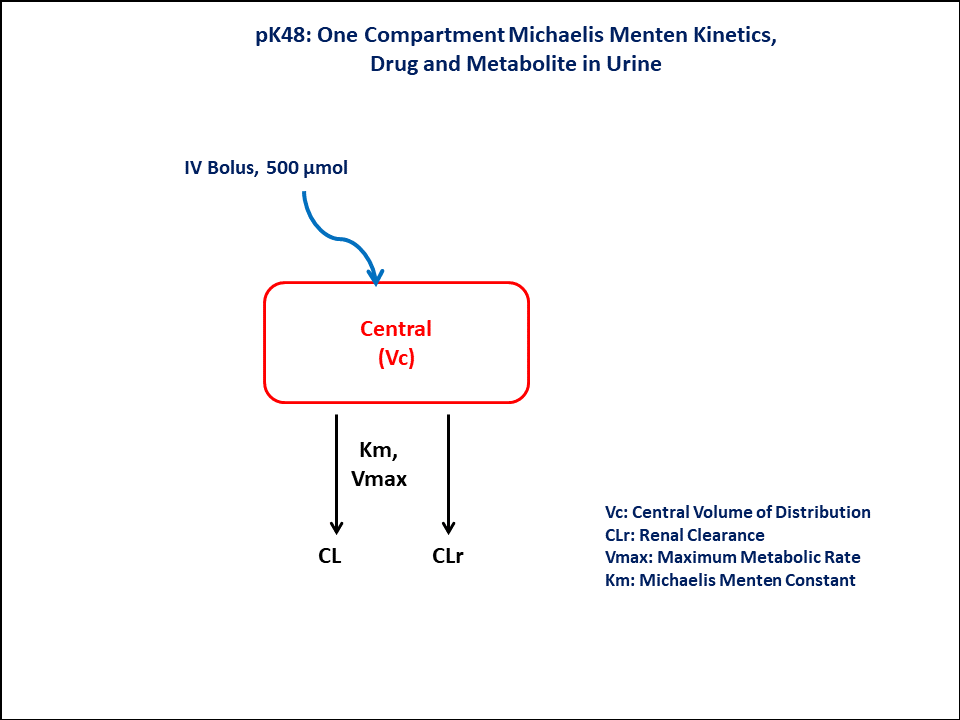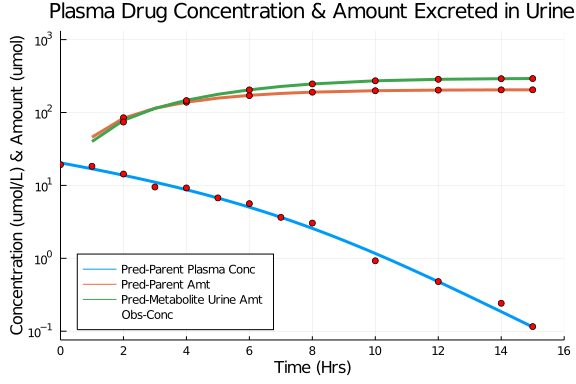# Exercise PK48 - One Compartment Michaelis Menten Kinetics, Drug and metabolite in Urine

### Background

• Structural model - One Compartment Michaelis Menten Kinetics, Drug and metabolite in Urine

• Route of administration - IV bolus

• Dosage Regimen - 500 micromol IV

• Number of Subjects - 1### Learning Outcome

In this model, you will learn -

• To build One Compartment model for the drug given Intravenous Bolus dosage, following Michaelis Menten Kinetics.

• To estimate the fundamental parameters involved in building the model.

• To apply differential equation in the model as per the compartment model.

• To design the dosage regimen for the subjects and simulate the plot.

### Objectives

In this tutorial, you will learn how to build One Compartment Michaelis Menten Kinetics, with Drug and Metabolite in Urine

### Libraries

call the "necessary" libraries to get started

using Pumas
using Plots
using CSV
using StatsPlots
using Random


### Model

In this One compartment model, we administer dose on Central compartment.

pk_48        = @model begin
@param begin
tvvmax   ∈ RealDomain(lower=0)
tvkm     ∈ RealDomain(lower=0)
tvclr    ∈ RealDomain(lower=0)
tvvc     ∈ RealDomain(lower=0)
Ω        ∈ PDiagDomain(4)
σ²_prop  ∈ RealDomain(lower=0)
end

@random begin
η        ~ MvNormal(Ω)
end

@pre begin
Vmax     = tvvmax * exp(η)
Km       = tvkm * exp(η)
Clr      = tvclr * exp(η)
Vc       = tvvc * exp(η)
end

@vars begin
VMKM    := Vmax*(Central/Vc)/(Km + (Central/Vc))
end

@dynamics begin
Central' = -VMKM - (Clr/Vc)* Central
UrineP'  = (Clr/Vc) * Central
UrineM'  =  VMKM
end

@derived begin
cp       = @. Central/Vc
ae_p     = @. UrineP
ae_m     = @. UrineM
dv       ~ @. Normal(cp, sqrt(cp^2*σ²_prop))
dv_aep   ~ @. Normal(ae_p, sqrt(cp^2*σ²_prop))
dv_aem   ~ @. Normal(ae_m, sqrt(cp^2*σ²_prop))
end
end

PumasModel
Parameters: tvvmax, tvkm, tvclr, tvvc, Ω, σ²_prop
Random effects: η
Covariates:
Dynamical variables: Central, UrineP, UrineM
Derived: cp, ae_p, ae_m, dv, dv_aep, dv_aem
Observed: cp, ae_p, ae_m, dv, dv_aep, dv_aem


### Parameters

The parameters are as given below. tv represents the typical value for parameters.

• Vmax - Maximum rate of metabolism (uM/hr)

• Km - Michaelis Menten Constant (uM)

• Clr - Renal Clearance (L/hr)

• Vc - Central Volume of Distribution (L)

• Ω - Between Subject Variability

• σ - Residual error

param = (tvvmax    = 51.4061,
tvkm      = 5.30997,
tvclr     = 2.46764,
tvvc      = 24.5279,
Ω         = Diagonal([0.0,0.0,0.0,0.0]),
σ²_prop   = 0.02)

(tvvmax = 51.4061, tvkm = 5.30997, tvclr = 2.46764, tvvc = 24.5279, Ω = [0.
0 0.0 0.0 0.0; 0.0 0.0 0.0 0.0; 0.0 0.0 0.0 0.0; 0.0 0.0 0.0 0.0], σ²_prop
= 0.02)


### Dosage Regimen

Intravenous bolus dosing of 500 micromol to a single subject at time=0.

ev1    = DosageRegimen(500, cmt=1, time=0)
sub1   = Subject(id=1, events=ev1)

Subject
ID: 1
Events: 1


### Simulation & Plot

Lets simulate for plasma concentration with the specific observation time points after IV bolus dose.

Random.seed!(123)
sim_sub1 = simobs(pk_48,sub1,param,obstimes=0:0.1:15)
df1      = DataFrame(sim_sub1)


### Dataframe & Plots

Use the dataframe for plotting

• cp - Predicted drug concentration in plasma

• ae_p - Predicted amount excreted in urine (Parent)

• ae_m - Predicted amount excreted in urine (Metabolite)

• dv - Observed drug concentration in plasma

• dv_aep - Observed amount excreted in urine (Parent)

• dv_aem - Observed amount excreted in urine (Metabolite)

df1_dv = filter(x -> x.time in [0,1,2,3,4,5,6,7,8,10,12,14,15], df1)
df2_dv = filter(x -> x.time in [2,4,6,8,10,12,14,15], df1)

@df df1 plot(:time, :cp, yaxis=:log, legend=:bottomleft, linewidth=3,
label="Pred-Parent Plasma Conc", title="Plasma Drug Concentration & Amount Excreted in Urine",
xlabel= "Time (Hrs)", ylabel="Concentration (umol/L) & Amount (umol)",
xlims=(0,16), xticks=[0,2,4,6,8,10,12,14,16], ylims=[0.1,1000])
@df df1_dv plot!(:time, :ae_p, label="Pred-Parent Amt", linewidth=3 )
@df df1_dv plot!(:time, :ae_m, label="Pred-Metabolite Urine Amt",linewidth=3)
@df df1_dv scatter!(:time, :dv, label="Obs-Conc", color=:red)
@df df2_dv scatter!(:time, :dv_aep, color=:red, label=false)
@df df2_dv scatter!(:time, :dv_aem, color=:red, label=false)